• ## Calculating a depreciating asset's decline in value after its effective life has been recalculated

If you are using the diminishing value method to calculate the decline in value of the asset, the new estimate of effective life is used in the diminishing value formula: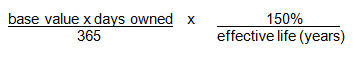if you start to hold the asset prior to 10 May 2006, or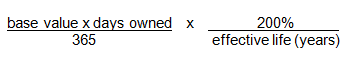if you start to hold the asset on or after 10 May 2006.

Under the prime cost method, an adjusted prime cost formula must be used from the year in which the effective life is recalculated: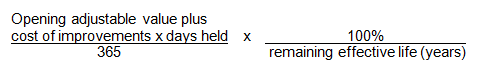In the adjusted formula, the asset's opening adjustable value (plus the cost of any improvements made to the asset during the year) is substituted for the asset's cost. For effective life, you substitute the period of the recalculated effective life that remains at the start of the year in which you make the new estimate.

Example (diminishing value method)

Using the facts of the previous example, assume that DA Pty Ltd chose to use the diminishing value method to calculate the decline in value of the asset and the cost of the asset is \$10,000. The company's deduction for 2001–02 would be \$2,143 calculated as follows: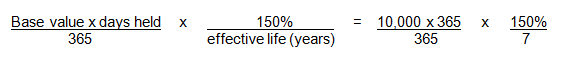For 2002–03, the company recalculates the effective life for the asset at two years. The asset's opening adjustable value for 2002–03 is \$7,857 (that is, \$10,000 less \$2,143). DA Pty Ltd can claim depreciation of \$5,893 for the year, based on the new estimate of effective life of two years as follows: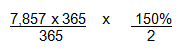Example (prime cost method)

Assume that DA Pty Ltd uses the prime cost method to calculate the decline in value of the asset. For 2001–02, the deduction for the decline in value of the asset would be \$1,429 calculated as follows: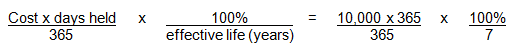For 2002–03 the company recalculates the effective life for the asset at two years.

Under the prime cost method, an adjusted formula must be used to calculate the decline in value for this and later years. The asset's remaining effective life, worked out as at the start of the income year, is one year. This figure is used instead of the earlier estimate of the asset's effective life and the opening adjustable value of \$8,571 (that is, \$10,000 less \$1,429) is substituted for the asset's cost. The deduction for 2002–03 would be \$8,571 calculated as follows: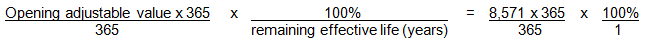End of example